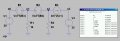# Help, finding current and voltage using Kirchhoff's Law

#### Luan Cristian Thums

Joined Aug 29, 2014
6
What I tried, so far, is using both KLs, ended up with a bunch of equations and found I1 = -0.0367A, I2 = -2.94A and I3 = -3.19A. What I'm supposed to find are the currents I1, I2, I3 and the voltages V1 and V2.

Can someone point me in the right direction here? What is the best approach to solve this problem?

I'm attaching a image of the circuit.

Thank you.

#### wmodavis

Joined Oct 23, 2010
739
Write three loop equations.
Use standard mathematical techniques to solve for the unknowns I1, I2 & I3.
With those you can use Ohms law to determine the voltage drops V1 & V2 across the two resistors.

•Luan Cristian Thums

#### Jony130

Joined Feb 17, 2009
5,241
Notice that V1 = 20V + 7*I3 , and I3 = 5*V1
So form there we can find V1 and I3
V1 = 20V + 7*5*V1 ---->V1 = -20/34 = -0.588235V
And I3 = 5*V1 = 2.9411A

And V2 = V1 + 2*I3

•Luan Cristian Thums

#### shteii01

Joined Feb 19, 2010
4,644

#### Luan Cristian Thums

Joined Aug 29, 2014
6

Sorry about that, it most certainly could have been resized.

#### JoeJester

Joined Apr 26, 2005
4,390
here's a more reasonable size ....

#### Attachments

•Luan Cristian Thums

#### eduardo schmoller

Joined Sep 23, 2014
1
I found: I1 = -0.04 A, I2 = -2.94 A, I3 = 0.22 A, |V1| = 0.64 V, |V2| = 6,6 V

#### Jony130

Joined Feb 17, 2009
5,241
Last edited:

#### MikeML

Joined Oct 2, 2009
5,444
Last edited:
•Luan Cristian Thums

#### The Electrician

Joined Oct 9, 2007
2,846
Last edited:
•Luan Cristian Thums

#### Luan Cristian Thums

Joined Aug 29, 2014
6
Thank you sir, I got the same results!

Noticing that I3 = 5V1 and knowing that V1 = 20 + 7I3 I found I3 and V1, to later derive V2 from V2 = V1 - 2I3 (second loop).
Having the voltage drop on both resistors and knowing their resistance I used Ohm's law to get both still unkowned currents.

Notice that V1 = 20V + 7*I3 , and I3 = 5*V1
So form there we can find V1 and I3
V1 = 20V + 7*5*V1 ---->V1 = -20/34 = -0.588235V
And I3 = 5*V1 = 2.9411A

And V2 = V1 + 2*I3
I believe that V2 = V1 - 2I3

#### MikeML

Joined Oct 2, 2009
5,444

#### Luan Cristian Thums

Joined Aug 29, 2014
6
There is 2 basics answers on this thread (I believe?!) one coming from V2 = V1+2I3 and the other from V2 = V1-2I3.
Which one is correct?

#### Luan Cristian Thums

Joined Aug 29, 2014
6
Thank you very much for your help, specially for introducing me to this software. But R on the second resistor is 30 ohms.

#### Jony130

Joined Feb 17, 2009
5,241
There is 2 basics answers on this thread (I believe?!) one coming from V2 = V1+2I3 and the other from V2 = V1-2I3.
Which one is correct?
Well because 2I3 is connect backwards the correct one is V2 = V1-2I3

•Luan Cristian Thums

#### MikeML

Joined Oct 2, 2009
5,444
Thank you very much for your help, specially for introducing me to this software. But R on the second resistor is 30 ohms.
Also fixed:

#### The Electrician

Joined Oct 9, 2007
2,846
View attachment 73351

Here is what LTSpice says:
In your simulation, I(B3) has a different polarity than I(V3); this will give different results for those places that have a defining equation involving I3 as defined in the original circuit. It looks to me like the current direction of B3 is the one that agrees with I3.

For example, your defining equation for the voltage source B1 is 7*I(V3), but I(V3) is going in the opposite direction compared to I3.

What do you get if you change the definitions of B1 and B2 so they use I(B3) rather than I(V3)?

#### MikeML

Joined Oct 2, 2009
5,444
In your simulation, I(B3) has a different polarity than I(V3); this will give different results for those places that have a defining equation involving I3 as defined in the original circuit. It looks to me like the current direction of B3 is the one that agrees with I3.

For example, your defining equation for the voltage source B1 is 7*I(V3), but I(V3) is going in the opposite direction compared to I3.
The current in B3 and V3 is going in the same direction. LTSpice's convention (conventional current, not electron current) is that the current that flows out of the + terminal of a voltage source carries a negative sign. See the attached circuit:Since V3 is inverted, then -(I(V3)) is positive.

What do you get if you change the definitions of B1 and B2 so they use I(B3) rather than I(V3)?
A radically different (but correct) solution. This is because of the way that LTSpice handles Behavioral Voltage and Current sources.#### The Electrician

Joined Oct 9, 2007
2,846
In post #9, you've shown an LTSpice simulation, but you didn't say whether you believe it's correct. Do you?

Looking at the original circuit, the left hand mesh shows that V1 = 20 + 7*I3. The right hand mesh shows that I3 = 5*V1. Making the obvious substitution, we get V1 = 20 + 5*7*V1; from this we get V1 = -20/34=-.588235

This is so simple that surely it is not mistaken, but this isn't what LTSpice gets in post #9. Is there something I'm not understanding?